# Non Verbal Reasoning - Cubes and Dice

### Exercise :: Cubes and Dice - Section 1

The sheet of paper shown in the figure (X) given on the left hand side, in each problem, is folded to form a box. Choose from amongst the alternatives (1), (2), (3) and (4), the boxes that are similar to the box that will be formed.

16.

Choose the box that is similar to the box formed from the given sheet of paper (X).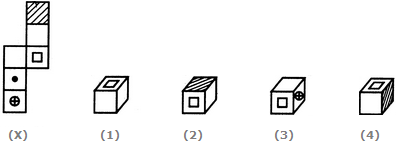A. 1 only B. 2 and 3 only C. 1 and 3 only D. 1, 2 and 4 only

Explanation:

The fig. (X) is similar to Form V. So, when the sheet shown in fig. (X) is folded to form a cube, then the face with shading lies opposite to the free bearing a square, the face bearing a dot lies opposite to a blank face and the face bearing a circle (with a '+' sign inside it) lies opposite to another blank face. The cubes in figures (2) and (4) have the shaded face adjacent to the face bearing a square. Therefore, the cubes in these two figures cannot be formed. Hence, only cubes in figures (1) and (3) can be formed.

17.

Which of the following finished patterns can be obtained from the piece of cardboard (X) shown below?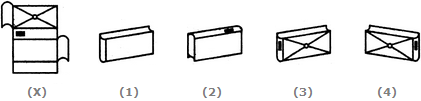A. 1 B. 2 C. 3 D. 4

Explanation:

The pattern on fig. (X) and also the fact that the faces are rectangle, indicate that only fig. (1) can be obtained by folding fig. (X).

18.

Choose the box that is similar to the box formed from the given sheet of paper (X).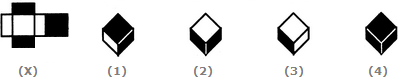A. 1 and 3 only B. 2 and 4 only C. 3 and 4 only D. 1 and 4 only

Explanation:

The fig. (X) is similar to Form I. So, when the sheet shown in fig. (X) is folded to form a box (cuboid), then the two rectangular-shaded faces lie opposite to each other, two rectangular white faces lie opposite to each other and the two square shaped faces (one shaded and one white) lie opposite to each other. Clearly, the cuboids shown in figures (2) and (4) cannot be formed as in each of the two cuboids the two shaded rectangular faces appear adjacent to each other. So, only the cuboids in figures (1) and (3) can be formed.

19.

Choose the box that is similar to the box formed from the given sheet of paper (X).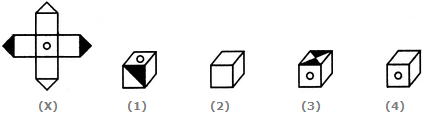A. 1 only B. 2 only C. 3 only D. 4 only

Explanation:

The fig. (X) is similar to the Form VI. So, when the cube is formed by folding the sheet shown in fig. (X), then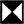is one of the faces of the cube and this face lies opposite to the face bearing a circle. Also, one of the blank faces lies opposite to another blank face and yet another blank face lies opposite to the fourth blank face. Thus, out of the four blank faces, no three faces can appear adjacent to each other.

Clearly, the cube in fig. (1) cannot be formed since there is no face of the type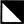, the cube in fig. (2) cannot be formed since it shows three blank faces adjacent to each other and the cube in fig. (3) cannot be formed since the face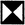cannot appear adjacent to the face bearing the circle. Hence, only the cube in fig. (4) can be formed.

20.

When the following figure is folded to form a cube, how many dots lie opposite the face bearing five dots?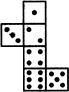A. 1 B. 2 C. 3 D. 4